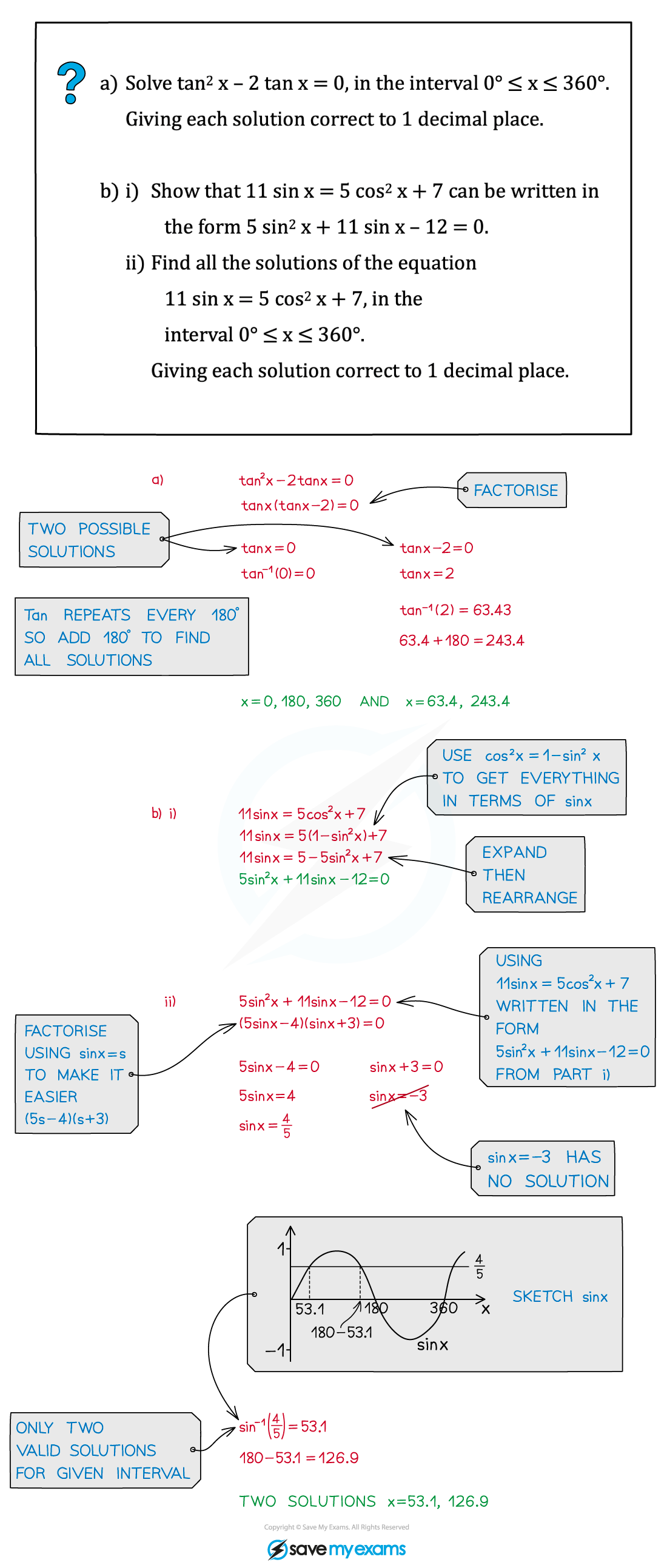# AQA A Level Maths: Pure复习笔记5.3.3 Quadratic Trigonometric Equations

### Quadratic Trigonometric Equations

#### Solving quadratic trigonometric equations

• If an equation involves sin2θ or cos2θ then it is a quadratic trigonometric equation
• These can be solved by factorising and/or using trigonometric identities (see Trigonometry – Simple Identities)
• As a quadratic can result in two solutions, will need to consider whether each solution exists and then find all solutions within a given interval for each#### Exam Tip

• Sketch the appropriate sin, cos, tan graph to ensure you find ALL solutions within the given interval, and be super-careful if you get a negative solution but have a positive interval.
• For example, for an equation, in the interval 0° ≤ x ≤ 360°, with solution sin x = ‑¼ then sin‑1(‑¼) = -14.5 (to 1d.p.), which is not between 0 and 360 – by sketching the graph you’ll be able to spot the two solutions will be 180 + 14.5 and 360 ‑ 14.5.

#### Worked Example